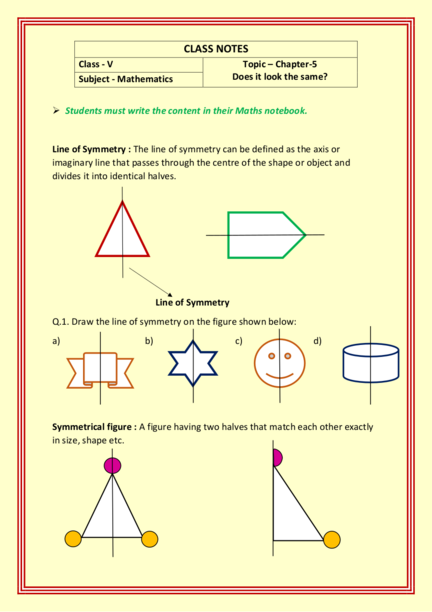# Line of Symmetry and Rotation of FiguresContributed by:In this pdf, we will see the lines of symmetry. A line of symmetry is a line that cuts a shape exactly in half. This means that if you were to fold the shape along the line, both halves would match exactly. Equally, if you were to place a mirror along the line, the shape would remain unchanged.
1. CLASS NOTES
Class - V Topic – Chapter-5
Subject - Mathematics Does it look the same?
 Students must write the content in their Maths notebook.
Line of Symmetry : The line of symmetry can be defined as the axis or
imaginary line that passes through the centre of the shape or object and
divides it into identical halves.
Line of Symmetry
Q.1. Draw the line of symmetry on the figure shown below:
a) b) c) d)
Symmetrical figure : A figure having two halves that match each other exactly
in size, shape etc.
2. (By folding the above shape along the symmetrical line, one half will cover the
other similar half completely. So the two here are mirror halves.)
Q.2. Write all the alphabets of English in capital letters which have line of
symmetry.
Ans. A B C D E H I K
M O T U V W X Y
Q.3. Write all the alphabets of English in capital letters, which do not have any
line of symmetry.
Ans. F G J L N P Q R S
Z
Q.4. Put ‘✓’ for the shapes which can be divided into mirror halves and
‘✗’ for the shapes which cannot be divided into mirror halves.
(✗) (✗) (✓)
(✓) (✗) (✗)
*In standard-V, we are taking care of colour also before deciding the symmetry of the figure.
3. Reflection : To reflect a shape means to produce its mirror image. Every
reflection has a mirror line.
Ex : A reflection of an “R” is a backwards “Я”.
C|Ɔ 3|Ɛ |
Thus, the reflection is a transformation in which the direction of one axis is
Q.5. Write the letters which appear reversed in a mirror.
Ans :
Q.6. Write the letters which remain same in the mirror image.
4. Rotation : A rotation is a transformation that turns a figure about fixed point
called the centre of rotation. An object and its rotation are the same shape and
size, but the figures may be turned in different directions.
Rotation of a figure:
**The content is absolutely prepared at home.**